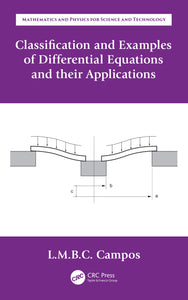# Classification and Examples of Differential Equations and their Applications

Regular price \$89.95 \$89.95 Sale

Author(s): Luis Manuel Braga da Costa Campos

Classification and Examples of Differential Equations and their Applications is the sixth book within Ordinary Differential Equations with Applications to Trajectories and Vibrations, Six-volume Set. As a set, they are the fourth volume in the series Mathematics and Physics Applied to Science and Technology. This sixth book consists of one chapter (chapter 10 of the set). It contains 20 examples related to the preceding five books and chapters 1 to 9 of the set. It includes two recollections: the first with a classification of differential equations into 500 standards and the second with a list of 500 applications.

The ordinary differential equations are classified in 500 standards concerning methods of solution and related properties, including: (i) linear differential equations with constant or homogeneous coefficients and finite difference equations; (ii) linear and non-linear single differential equations and simultaneous systems; (iii) existence, unicity and other properties; (iv) derivation of general, particular, special, analytic, regular, irregular, and normal integrals; (v) linear differential equations with variable coefficients including known and new special functions.

The theory of differential equations is applied to the detailed solution of 500 physical and engineering problems including: (i) one- and multidimensional oscillators, with damping or amplification, with non-resonant or resonant forcing; (ii) single, non-linear, and parametric resonance; (iii) bifurcations and chaotic dynamical systems; (iv) longitudinal and transversal deformations and buckling of bars, beams, and plates; (v) trajectories of particles; (vi) oscillations

and waves in non-uniform media, ducts, and wave guides.

• Provides detailed solution of examples of differential equations of the types covered in tomes l-5 of the set (Ordinary Differential Equations with Applications to Trajectories and Vibrations, Six -volume Set)
• Includes physical and engineering problems that extend those presented in the tomes 1-6 (Ordinary Differential Equations with Applications to Trajectories and Vibrations, Six-volume Set)
• Includes a classification of ordinary differential equations and their properties into 500 standards that can serve as a look-up table of methods of solution
• Covers a recollection of 500 physical and engineering problems and sub-cases that involve the solution of differential equations
• Presents the problems used as examples including formulation, solution, and interpretation of results

Review(s):

EAN: 9780429595158